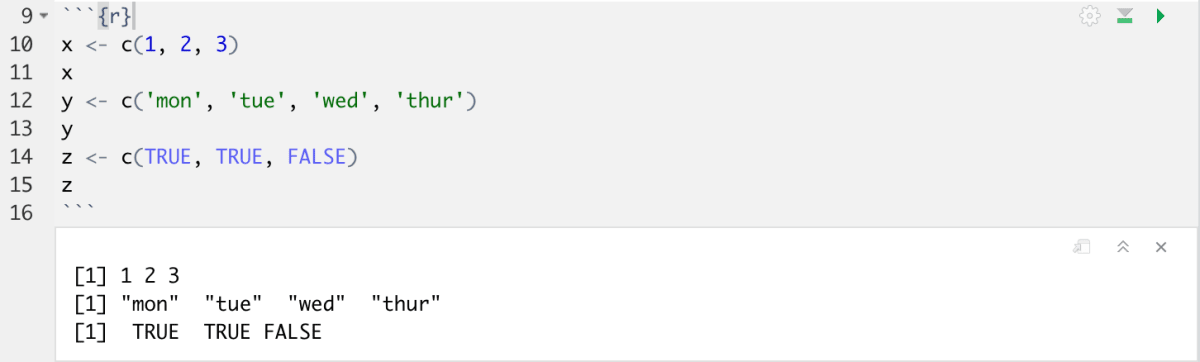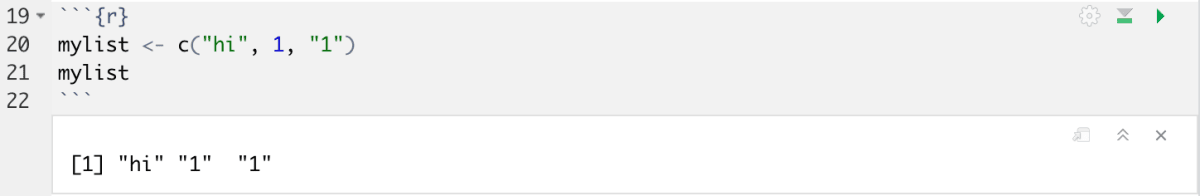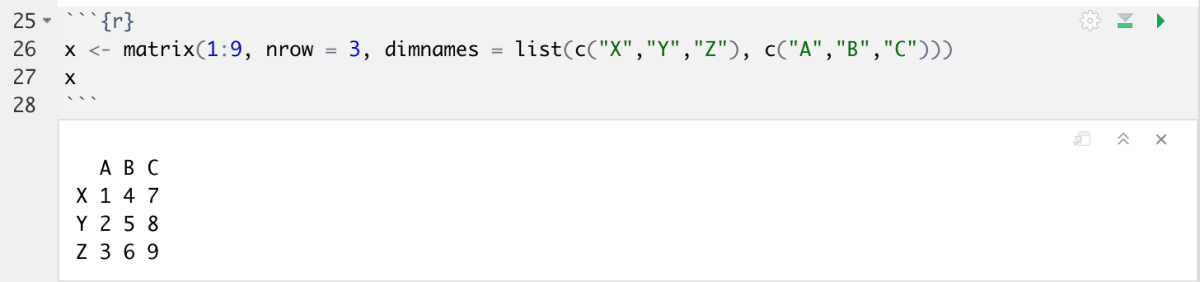Skip to content

# Data Structures

Data structures are a format of storing data that makes accessing and utilizing the data efficient. It is important to understand the syntax involved with these formats, as most other processes, such as data wrangling, will require the storage of data as a preliminary step.

## Vectors:

Vectors are ordered collections of values. They can be numeric, character, complex or logical values.

• Numeric:
• `x <- c(1, 2, 3)`
• Character:
• `y <- c('mon', 'tue', 'wed', 'thur')`
• Logical:
• `z <- c(TRUE, TRUE, FALSE)`

Try this out in your environment by pasting the code above.To print a variable, simply type the name of the variable. Note the new additions in your environment window as well!

## Lists:

The general form of vectors with different types of elements

`mylist <- c("hi", 1, "1")`## Matrices:

A matrix is a two dimensional data structure that contains elements of the same mode (i.e. numeric, character, etc).
This is a method of creating a matrix. The values in the matrix are specified with the first argument `1:9`. The dimensions and the names of the dimensions are specified in the second and third arguments.

```x <- matrix(1:9, nrow = 3, dimnames = list(c("X","Y","Z"), c("A","B","C")))
x```## Data Frames

Data frames are also two dimensional structures, but their values can be of different data types. `iris` is a data frame that comes with base R. Let’s take a look at the dataset. You can see that the dataset has both numbers and strings as datatypes.

`iris`## Factors:

By specifying some grouping information, you can modify and create special types of vectors using factors. In the example below, I’ve created two levels for the factor, complete and incomplete.

```x <- factor(c("complete","incomplete","complete","complete"))
str(x)```## Arrays:

Arrays are similar to matrices in that they are data structures with the same type of data. Arrays differ such that they can have more than two dimensions.

## Assignment in R:

When storing a data into a variable, you would have noticed the arrow mark `<-` being used. Let’s look at the syntax for assignment in R:

`x <- y`

X is a variable name and Y can be any data (data frame, vector, value etc)

In many other languages, the syntax for assigning a value to a variable looks like this `x = y`.  The arrow is considered to have precedence over the equal to sign in R.

Sticking to common practice, let’s use `x <- y` for assigning a value to a variable and `x = y` for assignment to arguments.

` variable <- function(arg1 = y)`